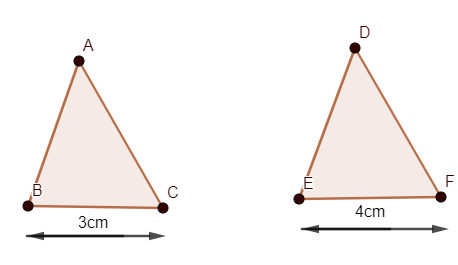Courses
Courses for Kids
Free study material
Free LIVE classes
MoreLIVE
Join Vedantu’s FREE Mastercalss

# If $\Delta ABC$ is similar to $\Delta DEF$ such that BC=3 cm, EF=4 cm and area of $\Delta ABC$=54$c{{m}^{2}}$. Determine the area of $\Delta DEF$.A. $40c{{m}^{2}}$B. $59c{{m}^{2}}$C. $69c{{m}^{2}}$D. $96c{{m}^{2}}$Verified
361.8k+ views
Hint: Use the property of similar triangles which is related to the area of triangles and sides of the similar triangles which is given as “the ratio of area of similar triangles is equal to the ratio of the square of sides.”

Here, we have two triangles $\Delta ABC$ and $\Delta DEF$ which are similar to each other. And side BC of $\Delta ABC$ is given as 3cm and side EF of $\Delta DEF$ is given as 4cm. And we need to determine the area of $\Delta DEF$ if the area of $\Delta ABC$ be $54c{{m}^{2}}$.Now, we need to use the property of similar triangles with respect to the area of them. Relation between sides and area of similar triangles can be given as
Area of triangle 1/Area of triangle 2=${{\left( \dfrac{\text{Side of triangle 1}}{\text{side of triangle 2}} \right)}^{2}}\ldots \ldots (1)$
So, by applying the above property with the similar triangles ABC and DEF, we get
$\dfrac{area(\Delta ABC)}{are(\Delta DEF)}={{\left( \dfrac{AB}{DE} \right)}^{2}}={{\left( \dfrac{BC}{EF} \right)}^{2}}={{\left( \dfrac{AC}{DF} \right)}^{2}}\ldots \ldots (2)$
Now we have values of sides BC and EF and area of $\Delta ABC$, so, by substituting values of BC, EF and area($\Delta ABC$),
We have,
$area(\Delta ABC)=54c{{m}^{2}}$
BC=3cm
EF=4cm
Hence,
\begin{align} & \dfrac{54}{area\left( \Delta DEF \right)}={{\left( \dfrac{3}{4} \right)}^{2}} \\ & \dfrac{54}{area\left( \Delta DEF \right)}=\dfrac{9}{16} \\ \end{align}
On cross-multiplying, we get
\begin{align} & area\left( \Delta DEF \right)=\dfrac{16\times 5}{9} \\ & area\left( \Delta DEF \right)=16\times 6=96c{{m}^{2}}. \\ \end{align}
Hence, the area of $\Delta DEF$can be given as $96c{{m}^{2}}$.
Therefore, option D is the correct answer.
Note: One can apply property of similar triangles as, $\dfrac{area(\Delta ABC)}{are(\Delta DEF)}=\dfrac{BC}{EF}$ which is wrong.
So, we need to take care with the relations of areas of similar triangles and their sides.
One can prove the given property by writing area of two similar triangles as
Area =$\dfrac{1}{2}\times base\times height$.
And use the property of similar triangles that the ratio of sides of similar triangles is equal.

Last updated date: 25th Sep 2023
Total views: 361.8k
Views today: 6.61k